# Colpitts oscillator PSPICE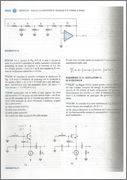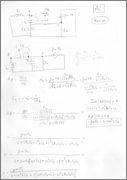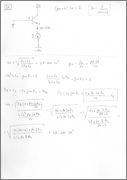Hi,
I'm trying to simulate exercise 13.21(a) from Sedra-Smith, with:

• Vcc=5V
• f=100 kHz
and the following BJT model:
Code:
.model modn NPN(Is=6.734f Xti=3 Eg=1.11 Vaf=74.03 Bf=416.4 Ne=1.259 Ise=6.734f Ikf=66.78m Xtb=1.5
Br=.7371 Nc=2 Isc=0 Ikr=0 Rc=1 Cjc=3.638p Mjc=.3085 Vjc=.75 Fc=.5 Cje=4.493p Mje=.2593 Vje=.75
Tr=239.5n Tf=301.2p Itf=.4 Vtf=4 Xtf=2 Rb=10)

This is the circuit in pspice: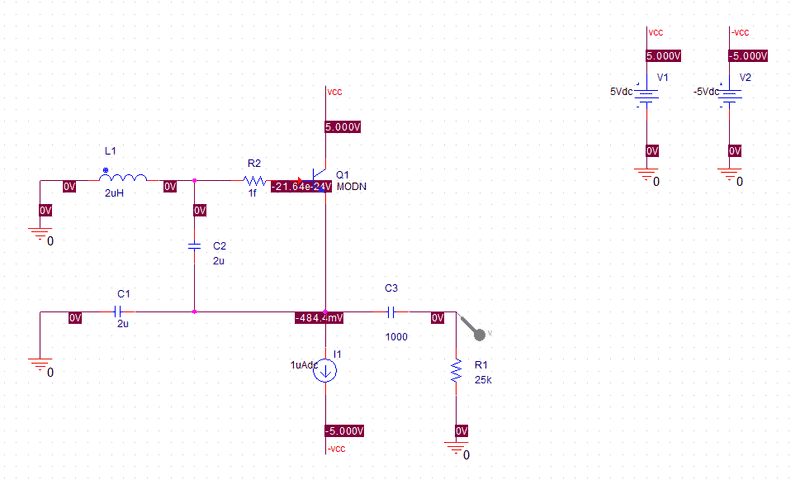(I had to put R2=1f because otherwise the simulation didn't converge)

Since I have two conditions for oscillation:

Code:
Im{A*B(jw)}=0
Code:
A*B(jw0)=1

but three electrical reactancesto size, then I have one degree of freedom so I chosed C1=L.

I also chosed I = 1*10^-6 A which should be fine because the BJT works in the forward-active region.

Unfortunaly when I simulate it I get very different results when I change the Run Time or Max Step Size values: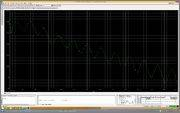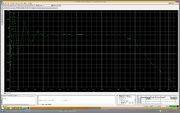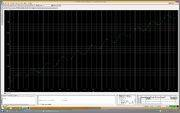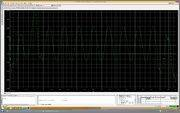What's wrong? :-(

This is the project file, including the models library if someone wants to try it:

Thanks

Svein
I do not know "Sedra-Smith", but I se several problems with your circuit.

1. The current source will take forever charge a 1000F capacitor (t =C3*V/I = 1000*5/1e-6 = 5e9 seconds or about 158years. You are trying for a frequency of about 700kHz, so go to a larger current and a much smaller capacitor (0.1μF should be large enough by far).

2. L and C are unbalanced. Try multiplying L with 10 and dividing C1 and C2 by 10.

Try this first and see what happens.

Finally I found the problem(s), there were many of them!

1) The biggest one were the initial conditions. After selecting "Skip the initial transient bias point calculation" (SKIPBP) in PSpice simulation's options everything got better.
2) Another big problem was in the math: I didn't size reactances well. I did it well, but just barely. In fact assuming A*B(jw0)=1 you barely get a persistent oscillation and a small rounding is enough to let it fade. So I assumed A*B(jw0)=100 when sizing reactances.
3) I had to put another small resistor in the project (R2=1f) because otherwise the simulation didn't converge, but it seems it was too small and I still had convergence problems in some circumstances. So I changed it to 1 mOhm instead.
4) The output capacitor took a long time to load (which wasn't a problem), but also lead to convergence problems in some circumstances. So I changed it to 1nF.
5) I also increased the current generator I1 to 1mA to get the oscillations sooner.
6) Finally I sized the reactances once again, assuming L=C1*10^3 to get more realistic values (but it works even if they are balanced).
7) Now THD is ~1% for the first 50 harmonics!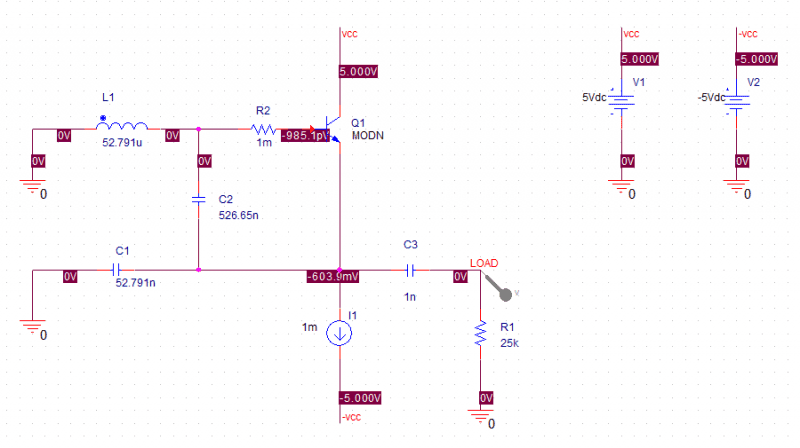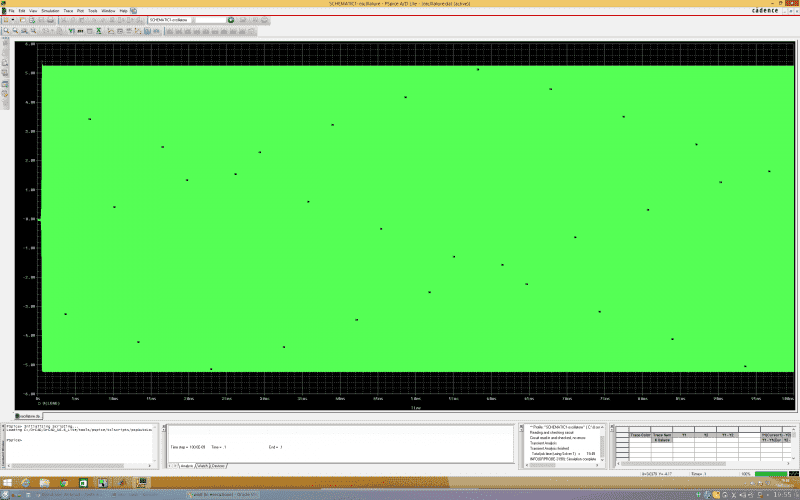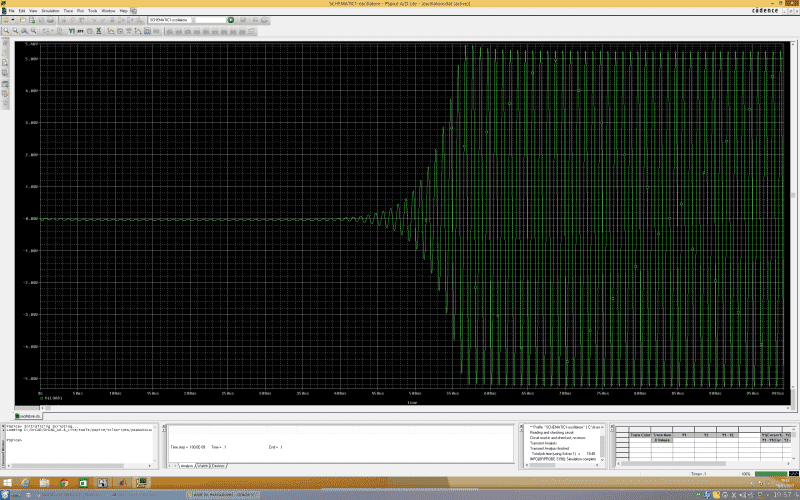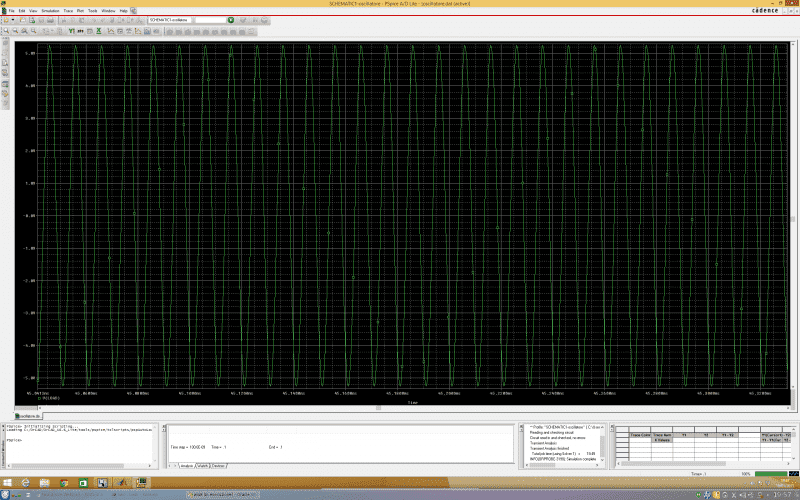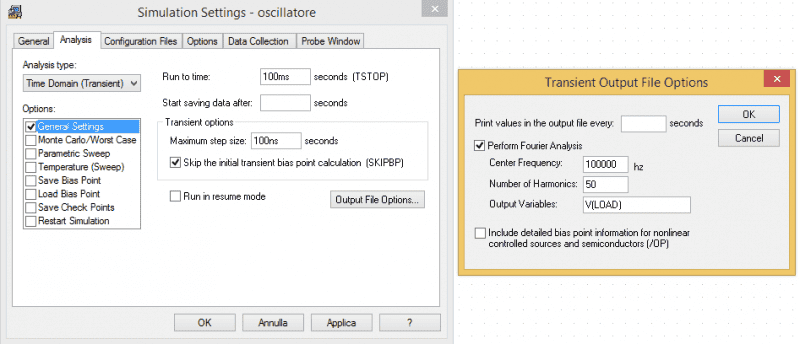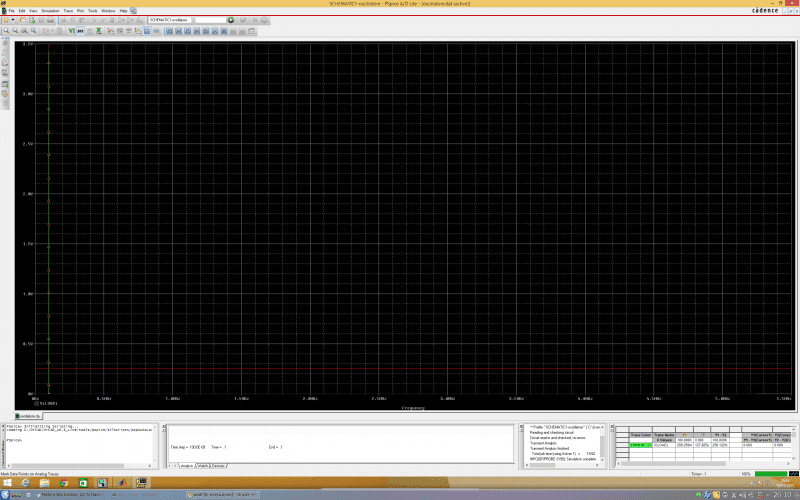Thanks for your help Svein, it is much apreciated!

Svein